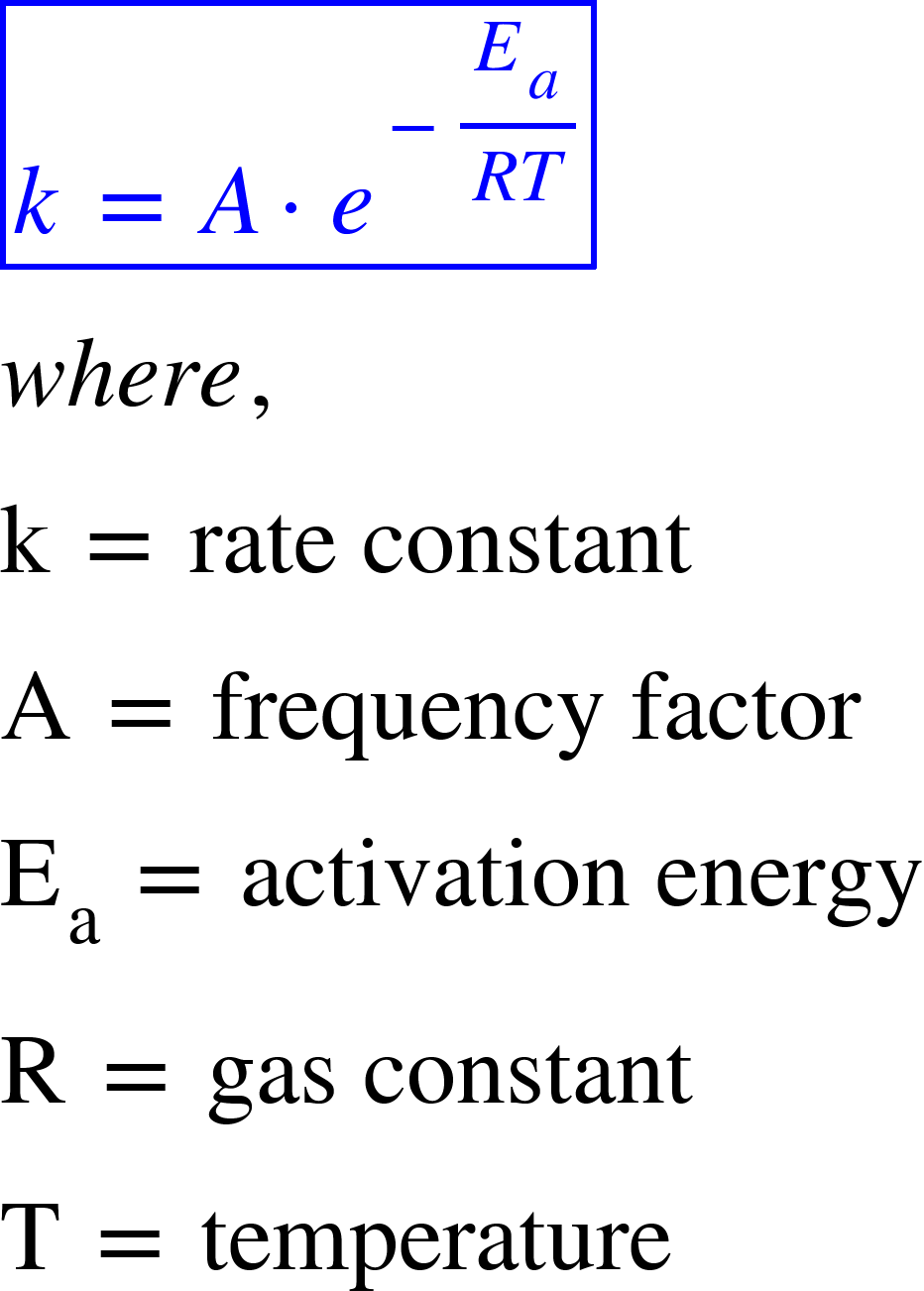# Problem: Reaction A and reaction B have identical frequency factors. However, reaction B has a higher activation energy than reaction A.Which reaction has a greater rate constant at room temperature?

###### FREE Expert Solution

The temperature dependence of a chemical reaction is represented by the Arrhenius equation:We have to determine whether reaction A or reaction B has a greater rate constant at room temperature while we are given that the activation energy for reaction B is higher.

95% (77 ratings)###### Problem Details

Reaction A and reaction B have identical frequency factors. However, reaction B has a higher activation energy than reaction A.

Which reaction has a greater rate constant at room temperature?